A*****m

O C T O B E R

O C T O B E R

O C T O B E R

###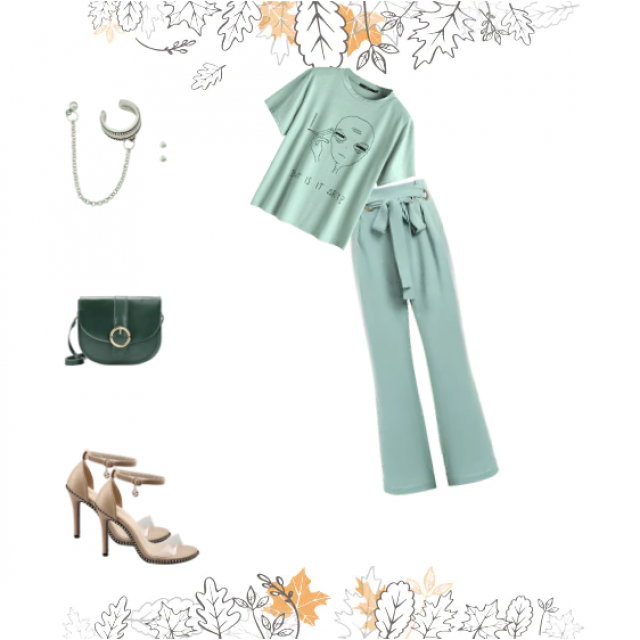S E P T E M B E R

[ outfit ]

###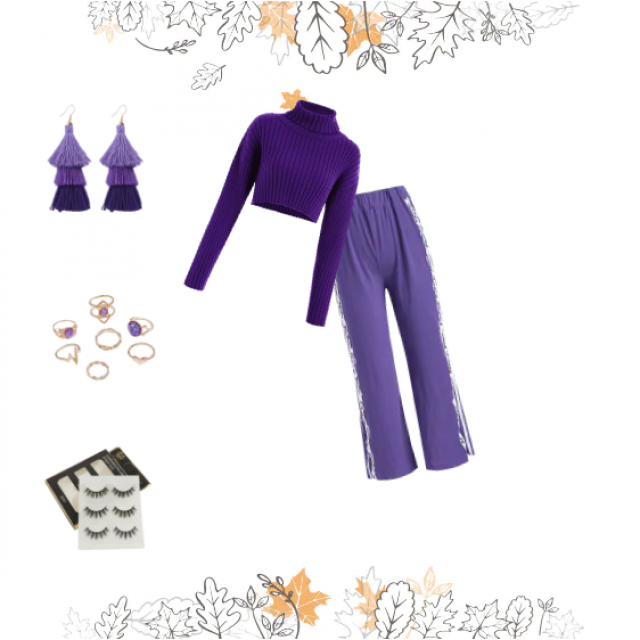S E P T E M B E R

[ outfit ]

###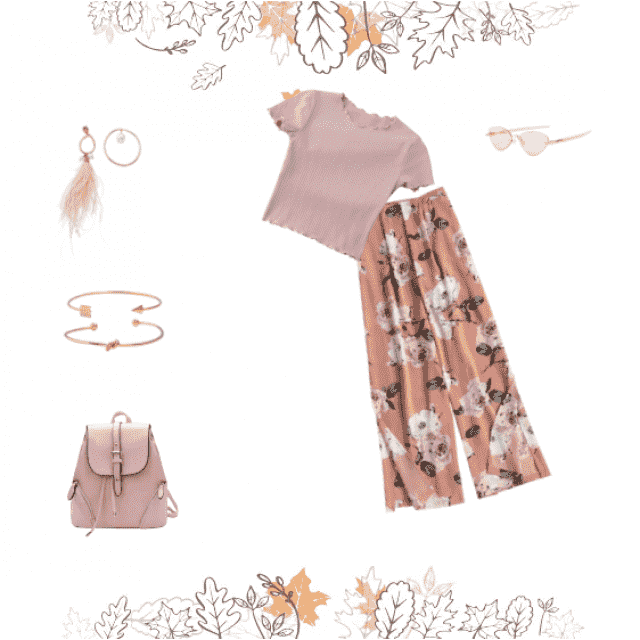S E P T E M B E R

[ outfit ]

###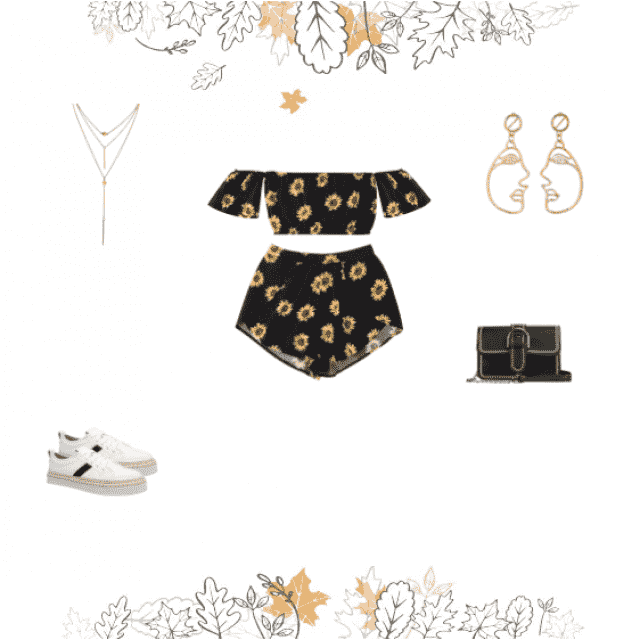S E P T E M B E R

[ outfit ]

###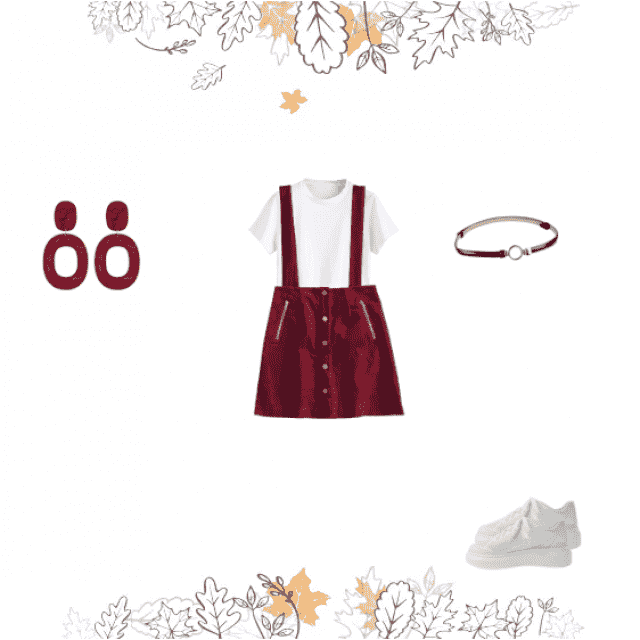S E P T E M B E R

[ outfit ]

###S E P T E M B E R

[ outfit ]

###S E P T E M B E R

[ outfit ]

###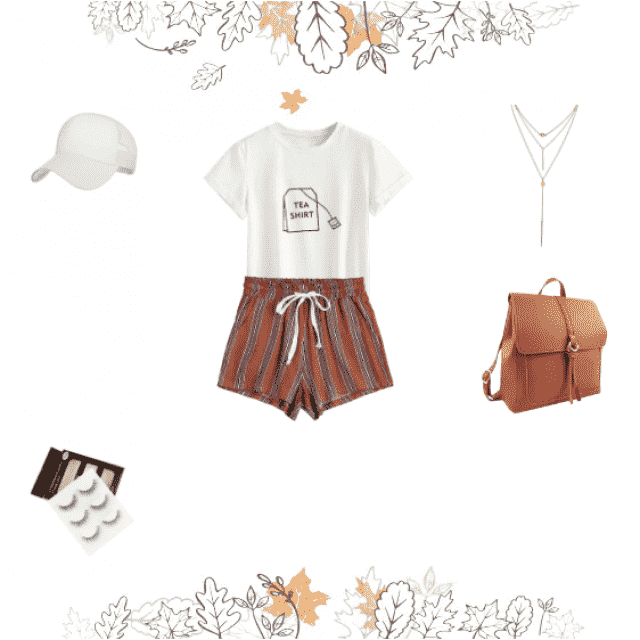S E P T E M B E R

[ outfit ]

###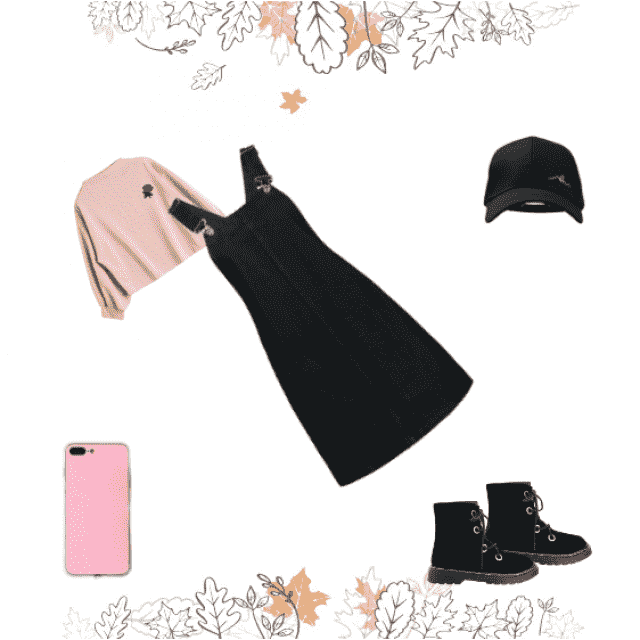S E P T E M B E R

[ outfit ]

S E P T EM B E R

[ outfit ]

###S E P T E M B E R

###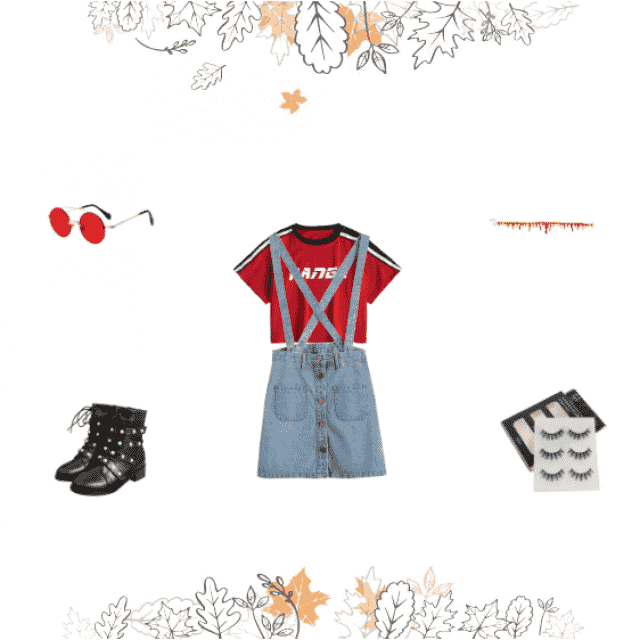S E P T E M B E R

[ outfit ]

###S E P T E M B E R

[ outfit ]

###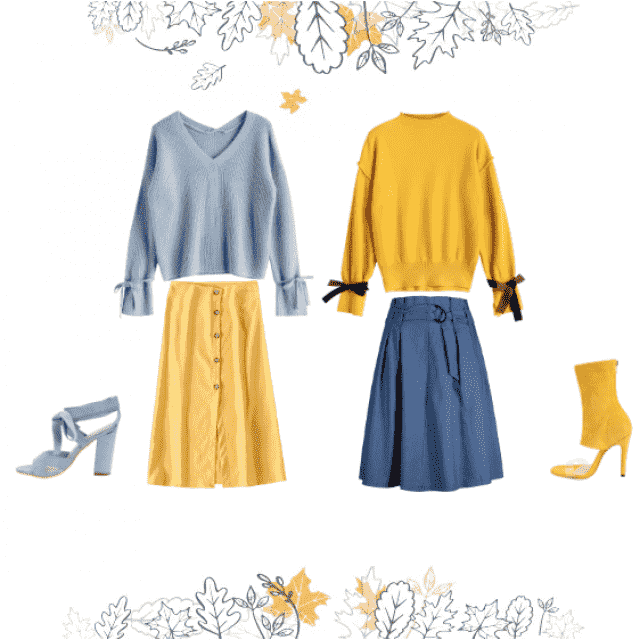S E P T E M B E R

###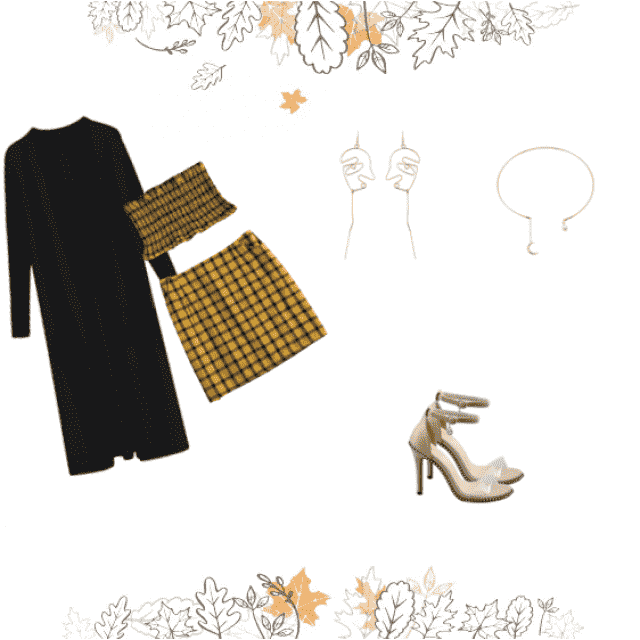S E P T E M B E R

[ outfit ]

###S E P T E M B E R

###S E P T E M B E R

[ outfit ]

Not any shows yet.

delete post×

Would you like to delete this post ?